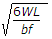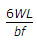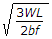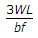# Civil Engineering - UPSC Civil Service Exam Questions

6.

Which of the following Pairs regarding explanations and the terminologies pertaining to masonry are correctly matched ?
1. Reveal....projecting stone to serve as support for joist.
2. Throating....groove provided on the underside of projecting elements likes sills.
3. Gable....triangle shaped masonry works provided at the ends of sloped roof.
4. Freeze....vertical sides of finished opening for doors and windows.
Select the correct answer using the codes given below :

 A. 1 and 2 B. 2 and 3 C. 3 and 4 D. 1 and 4

Explanation:

No answer description available for this question. Let us discuss.

7.

Which of the following are the purposes for use of steel bars reinforcement in cement concrete pavements ?
1. To increase the flexural strength of concrete
2. To prevent the onset of cracks
3. To allow wider spacing of joints.
Select the correct answer using the code given below :

 A. 1 and 2 only B. 2 and 3 only C. 1 and 3 only D. 1, 2 and 3

Explanation:

No answer description available for this question. Let us discuss.

8.

A simply supported beam of span 'L' carries a concentrated load 'W' at mid-span. If the width 'b' of the beam is constant and its depth is varying throughout the span, then what should be its mid-span depth, when design stress is 'f' ?

 A.B.C.D.Explanation:

No answer description available for this question. Let us discuss.

9.

The following readings were taken with a Dumpy level and a 4 m levelling staff on a continuously sloping ground at 30 m intervals : 0.680 m, 1.455 m, 1.855 m, 2.330 m, 2.885 m, 3.380 m, 1.055 m. The R.L. of the fourth point was calculated to be 79.100 m. The R.L. of the point that was read 0.680 m is

 A. 80.750 m B. 79.780 m C. 78.420 m D. 77.740 m

Explanation:

No answer description available for this question. Let us discuss.

10.

Consider the following statements :
1. In the laboratory consolidation test, initial compression is the result of displacement of soil particles.
2. Primary consolidation is due to dissipation of pore water pressure.
3. Secondary compression starts after complete dissipation of pore water pressure.
4. Primary consolidation and secondary compression occur simultaneously.
Which of the statements given above are correct ?

 A. 1 and 4 only B. 2 and 3 only C. 2 and 4 only D. 1 and 3 only